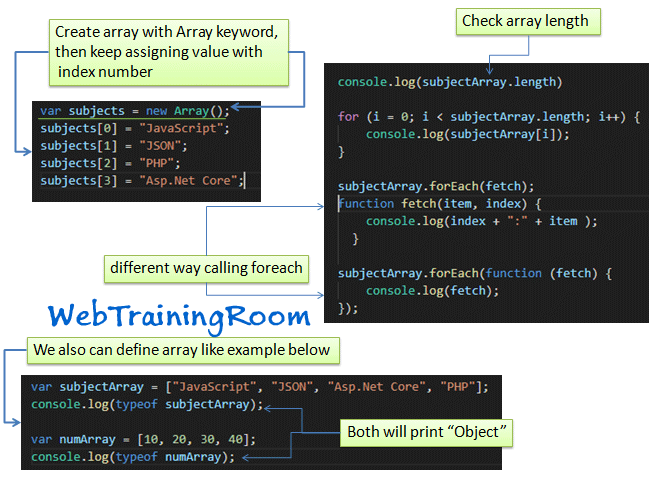# JavaScript Array Methods Example

In this tutorial you will learn how to work with Javascript Array!

To work with array we need to know JavaScript Array Iteration, JavaScript array object, JavaScript Array Methods etc, let's learn JavaScript Arrays tips, tricks with examples.An array is a special variable, can store multiple values in it.
Or you can say Array is a collection object of same type of data, or can be of different type of data, Array is very useful when we want to store big number of same type data and post or retrieve them in one call.

## How to Create Array in JavaScript?

Here we learn different aspect of working with usage of JavaScript array

Simple way of creating JavaScript Array using new keyword.
```<script>
var i;
var subjects = new Array(); //Creating array
subjects = "JavaScript";
subjects = "JSON";
subjects = "PHP";
subjects = "Asp.Net Core";
// Retrieving values from array
for (i = 0; i < subjects.length; i++) {
document.write(subjects[i] + "<br>");
}
</script>```

Store comma separated values in javaScript array example.

```<script>
var subjects = ["JavaScript", "JSON", "Asp.Net Core", "PHP"];
for (i = 0; i < subjects.length; i++) {
document.write(subjects[i] + "<br>");
}
</script>
```

If you want to get array length, you simply print subjects.length, means array variable .length

JavaScript Array Example

javascript Array Contains following methods

• JavaScript Array find
Javascript array find method

Array.find is an inbuilt function in JavaScript, Returns a value if element found in that array

```array.find(function(element))
```
```var memberAge = [13, 15, 18, 21];
var isValidAge = memberAge.find(function(age) {
return age >= 18;
});
// you also can write this way
function checkAge(age) {
return age >= 18;
}
var isValidAge = memberAge.find(checkAge)
```
• JavaScript Array push
javascript array push method

When you want to add element in javascript array, a new item in existing array, you can use push method. for example you want to add a new subject in above subjects array.

```var subjects = ["JavaScript", "JSON", "Asp.Net Core", "PHP"];
subjects.push("SQL Database");
var arrNumbers = [10, 20, 30, 40];
arrNumbers.push(60,70,80,90);
```
• JavaScript Array concat
Javascript array concat method

Concat method combines two or more arrays and returns a `new array();`

```var subjects = ["JavaScript", "JSON", "Asp.Net Core", "PHP"];
var subjects1 = ["Java", "Python", "LINQ", "XML"];
var subjects2 = subjects.concat(subjects1);
for (i = 0; i < subjects2.length; i++) {
document.writeln(subjects2[i] + "<br>");
}```
• JavaScript Array sort
Javascript array sort method

sort method is used to arrange the array elements in ascending order.

```var subjects = ["JavaScript", "JSON", "Asp.Net Core", "PHP"];
var subjects2 = subjects.sort();
for (i = 0; i < subjects2.length; i++) {
document.writeln(subjects2[i] + "");
}```
• JavaScript Array reverse
Javascript array reverse method

reverse method changes the sequence of elements in array and returns the reverse sequence

```var subjects = ["JavaScript", "JSON", "Asp.Net Core", "PHP"];
var subjects2 = subjects.reverse();
for (i = 0; i < subjects2.length; i++) {
document.writeln(subjects2[i] + "");
}```
• JavaScript Array forEach
Javascript array forEach method

`forEach()` method is used to invoke each item from specified array, we can call forEach in different ways

```var subjects = ["JavaScript", "JSON", "Asp.Net Core", "PHP"];

subjects.forEach(function (fetch) {
document.writeln(fetch);
});
// Or we can call a function like below
function fetch(item, index) {
console.log(index + ":" + item );
}
```
• JavaScript Array join
Javascript array join method

join method combines all the array elements into a string, you also can specify any separators, like any character you want to use in between

```<script>
var subjects = ["JavaScript", "JSON", "Asp.Net Core", "PHP"];
var subjects2 = subjects.join();
document.write(subjects2);
//using separators
var subjects3 = subjects.join('*');
document.write(subjects3);
</script>```
• JavaScript Array indexOf
Javascript array indexOf method

indexOf function is used for finding the index of the first occurrence of the any element (argument) in an Array.
indexOf will get the index position of search item, in following example we are finding the position of number 20

```<script>
var array = [10, 20, 30, 10,20];
var position = array.indexOf(20);
document.writeln(position);
// result will be 1
var position = array.indexOf(20, 2);
document.writeln(position);
// result will be 4
</script>```
Does JavaScript support two-dimensional array?

No, by default there is no way you can create two-dimensional array directly, however, you can create a two-dimensional array using logic like example below.

```let countries = [];

countries = [
['India','Delhi'],
['Australia','Canebera'],
['UK','London'],
];

console.table(countries)
```

Here is how the output will look like!

```┌─────────┬─────────────┬────────────┐
│ (index) │      0      │     1      │
├─────────┼─────────────┼────────────┤
│    0    │   'India'   │  'Delhi'   │
│    1    │ 'Australia' │ 'Canebera' │
│    2    │    'UK'     │  'London'  │
│    3    │  'Canada'   │ 'Toranto'  │
└─────────┴─────────────┴────────────┘
```

You may be interested to know how array is initiated in other programming language, look at following posts.

JavaScript programming tutorials to learn JavaScript coding step by step, develop website using JavaScript and html.
Javascript Array Function Examples
JavaScript Examples |